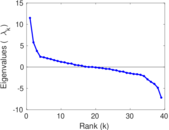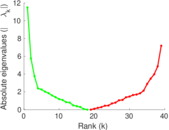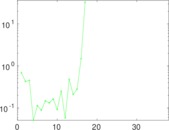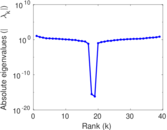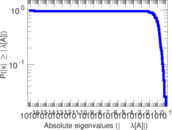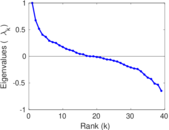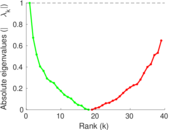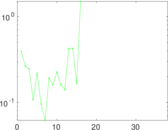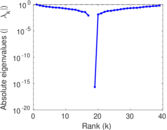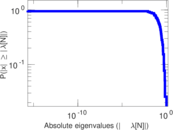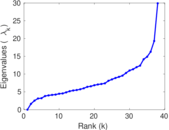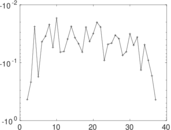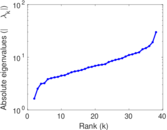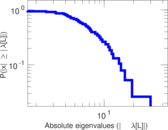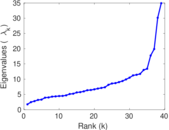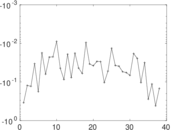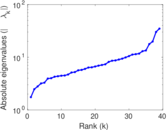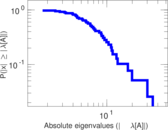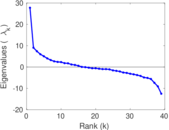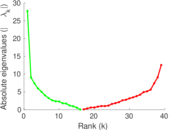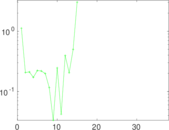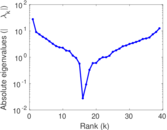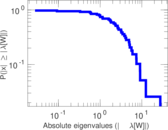# Chesapeake Bay

This is the mesohaline trophic network of Chesapeake Bay, an estuary in the United States of America. Nodes are groups of organisms such as phytoplankton or ciliates. Edges denote carbon exchange. The direction of exchange is not stored in this dataset. Neither are weights, i.e., amounts of exchange.

 Code `CB` Internal name `dimacs10-chesapeake` Name Chesapeake Bay Data source https://www.cc.gatech.edu/dimacs10/archive/clustering.shtml AvailabilityDataset is available for download Consistency checkDataset passed all tests Category Trophic network Dataset timestamp 1989 Node meaning Organism type Edge meaning Carbon exchange Network format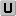Unipartite, undirected Edge typeUnweighted, no multiple edges LoopsDoes not contain loops Snapshot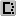Is a snapshot and likely to not contain all data Orientation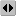Is not directed, but the underlying data is Multiplicity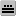Does not have multiple edges, but the underlying data has

## Statistics

 Size n = 39 Volume m = 170 Loop count l = 0 Wedge count s = 2,048 Claw count z = 12,393 Cross count x = 72,638 Triangle count t = 194 Square count q = 1,762 4-Tour count T4 = 22,628 Maximum degree dmax = 33 Average degree d = 8.717 95 Fill p = 0.229 420 Size of LCC N = 39 Diameter δ = 3 50-Percentile effective diameter δ0.5 = 1.395 12 90-Percentile effective diameter δ0.9 = 1.955 19 Median distance δM = 2 Mean distance δm = 1.828 63 Gini coefficient G = 0.317 496 Balanced inequality ratio P = 0.364 706 Relative edge distribution entropy Her = 0.949 334 Power law exponent γ = 2.106 65 Tail power law exponent γt = 3.571 00 Tail power law exponent with p γ3 = 3.571 00 p-value p = 0.625 000 Degree assortativity ρ = −0.375 783 Degree assortativity p-value pρ = 7.611 05 × 10−13 Clustering coefficient c = 0.284 180 Spectral norm α = 11.495 9 Algebraic connectivity a = 1.636 79 Spectral separation |λ1[A] / λ2[A]| = 1.599 44 Non-bipartivity bA = 0.374 781 Normalized non-bipartivity bN = 0.352 276 Algebraic non-bipartivity χ = 1.747 51 Spectral bipartite frustration bK = 0.050 112 5 Controllability C = 2 Relative controllability Cr = 0.051 282 1

## Plots

### Fruchterman–Reingold graph drawing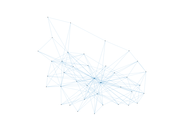### Degree distribution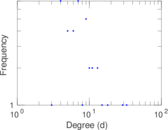### Cumulative degree distribution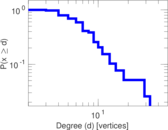### Lorenz curve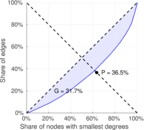### Spectral distribution of the adjacency matrix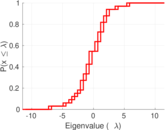### Spectral distribution of the normalized adjacency matrix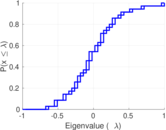### Spectral distribution of the Laplacian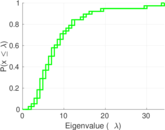### Spectral graph drawing based on the adjacency matrix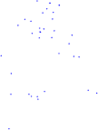### Spectral graph drawing based on the Laplacian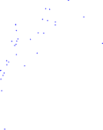### Spectral graph drawing based on the normalized adjacency matrix### Degree assortativity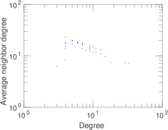### Zipf plot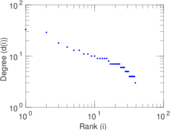### Hop distribution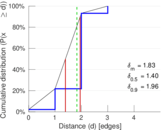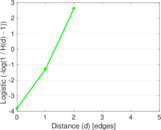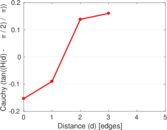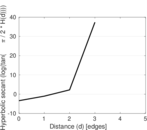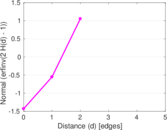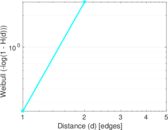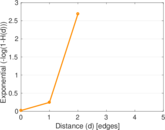### Double Laplacian graph drawing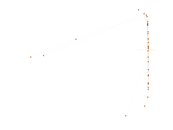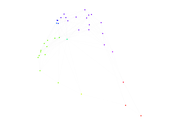### Delaunay graph drawing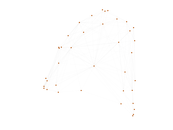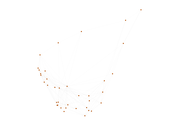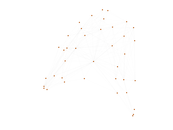### Clustering coefficient distribution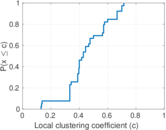### Average neighbor degree distribution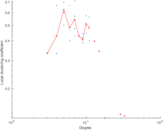### SynGraphy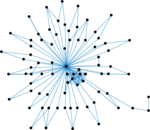### Matrix decompositions plots